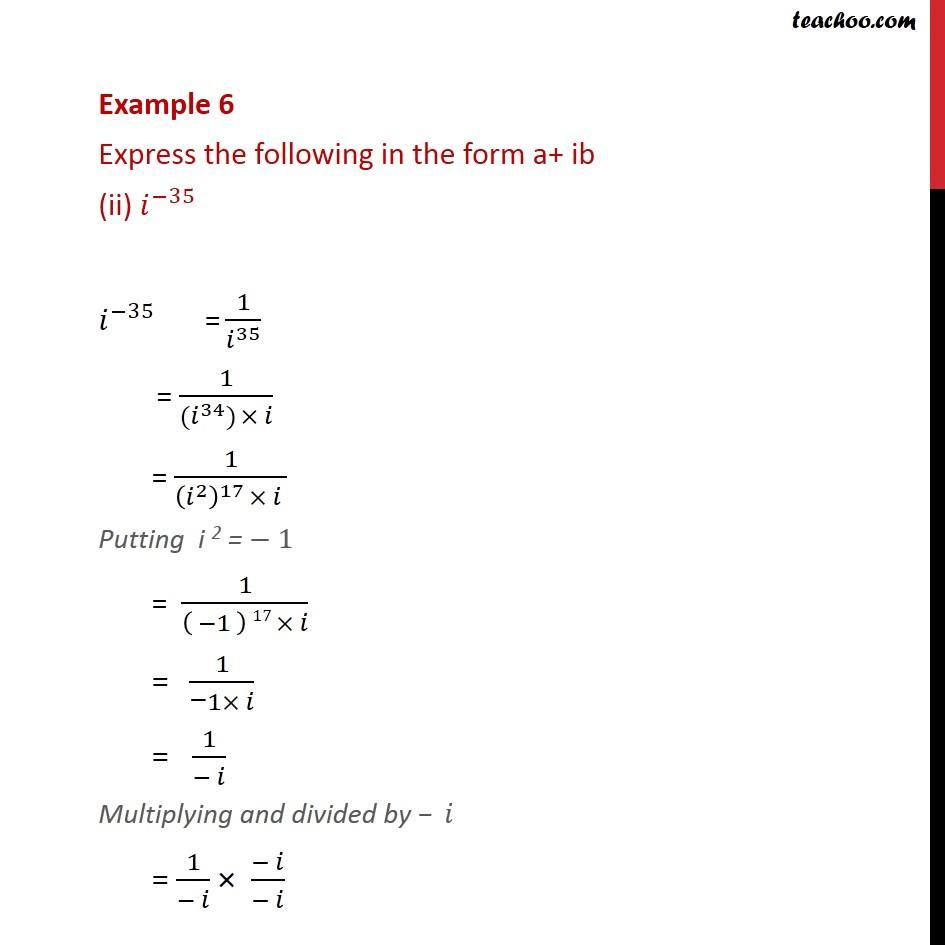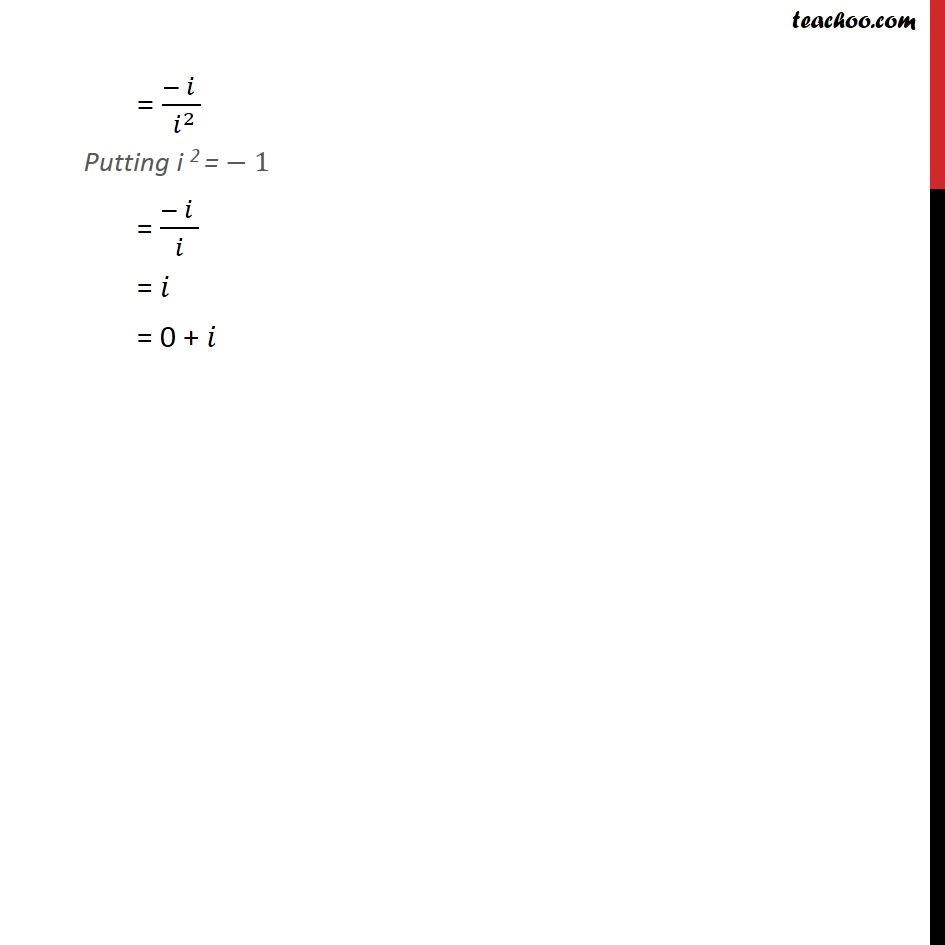Examples

Chapter 4 Class 11 Complex Numbers
Serial order wiseLearn in your speed, with individual attention - Teachoo Maths 1-on-1 Class

### Transcript

Example 6 Express the following in the form a+ ib (ii) ^( 35) ^( 35) = 1/ ^35 = 1/( ( ^34) ) = 1/(( ^2 )^17 ) Putting i 2 = 1 = 1/(( 1 ) 17 ) = 1/(" " 1 ) = 1/( ) Multiplying and divided by = 1/( ) ( )/( ) = ( )/( ^2 ) Putting i 2 = 1 = ( )/( ) = = 0 +